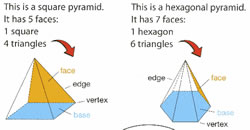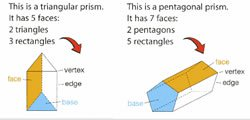# Difference between Pyramids and Prisms

##### Key difference: In geometry, pyramids and prisms are two different shapes. The main difference between a pyramid and prism is the fact that a prism has two bases, while the pyramid only has one.In geometry, pyramids and prisms are two different shapes. The main difference between a pyramid and prism is the fact that a prism has two bases, while the pyramid only has one.

A pyramid is a three-dimensional polyhedron. It has a base, which is a polygon. A polygon is any straight-sided shape, such as a triangle or a square. The base is connected to the sides or faces of the pyramid. The sides of the pyramid will always be triangular in shape. The sides of the pyramid meet the top. This is called the apex. Prisms are often used to show the dispersion of light into its constituent colors i.e the spectrum of light. Most of it has to do with the geometry and the refractive index of the material. Also, see the difference between line spectrum and band spectrum.

There are many types of pyramids, such as triangular pyramids, pentagonal pyramids, etc. The type of pyramid is determined by the base. For example: a triangular pyramid will have a triangular base, while a square pyramid will have a square base, and so on.

Also see: Difference between a parabola and hyperbola

A prism is also a three-dimensional polyhedron, but it looks very different from a pyramid. A prism has two identical ends and all flat sides. The sides or faces are perpendicular to the base faces, i.e. they make a right angle with the base. In case the sides are not perpendicular to the base it is called an oblique prism.

The prism is different than the pyramid in that it does not have an apex. Instead, it can be said that it has two bases. The bases are joined together by the sides. A way to differentiate the prism is to check whether the cross-section of the prism is the same all along its length.Similar to a pyramid, there are many types of prisms. The type of prism is determined by the shape of the base. For example, a triangular prism will have triangular bases, while a rectangular prism will have rectangular bases, an octagonal prism will have octagon bases, and so on.

The term prism has another connotation in optics. In optics, a prism is a piece of glass, crystal, or plastic that bends light. This happens as light moves slower in the prism than in the air. The prism splits the light into a spectrum. The light spectrum has the same colors as a rainbow. This refraction of light is the same phenomenon that causes rainbows.

An easy way to distinguish between a pyramid and a prism is to associate an image with each word. For example, the pyramids of Ancient Egypt are based on the geometric pyramid. Whereas a prism looks nothing like the pyramids of Ancient Egypt, it looks more like a tent or the shapes that game that toddlers play in which one has to sort prisms and insert them into holes.

Difference between Pyramids and Prisms:

 Pyramids Prisms Subject Geometry Geometry, Optics Type of Three-dimensional polyhedron Three-dimensional polyhedron Description A pyramid has a base, and all sides lead from the base to join at the top point. A prism has two identical ends and all flat sides. In optics, a prism is a piece of glass, crystal, or plastic that bends light causing light to appear in its rainbow spectrums. Number of Bases One Base Two Bases. The bases are joined together by the sides. Type of Bases Polygon Polygon Sides Sides are triangular in shape that meets at a point on the top called an apex. The sides or faces are perpendicular to the base faces, i.e. they make a right angle with the base. In case the sides are not perpendicular to the base it is called an oblique prism. Apex Has an apex Does not have an apex Types Many, such as triangular pyramids, pentagonal pyramids, etc. The type is determined by the shape of the base. For example, a triangular pyramid will have a triangular base Many, such as triangular prisms, pentagonal prisms, etc.  The type is determined by the shape of the base. For example, a triangular prism will have triangular bases Example The pyramids of Ancient Egypt The game where toddlers put blocks of shapes through the correct hole.

Image Courtesy: chatt.hdsb.ca

Thank u so much it helped me engineering drowning exam.

These figures are helped very much

In the 4th paragraph, you have the wrong 'whether': "A way to differentiate the prism is to check weather the cross section of the prism is the same all along its length."

First you should check your spelling. Whether is the correct one.

Thank you for pointing it out! It has been fixed.

Thanks for help......